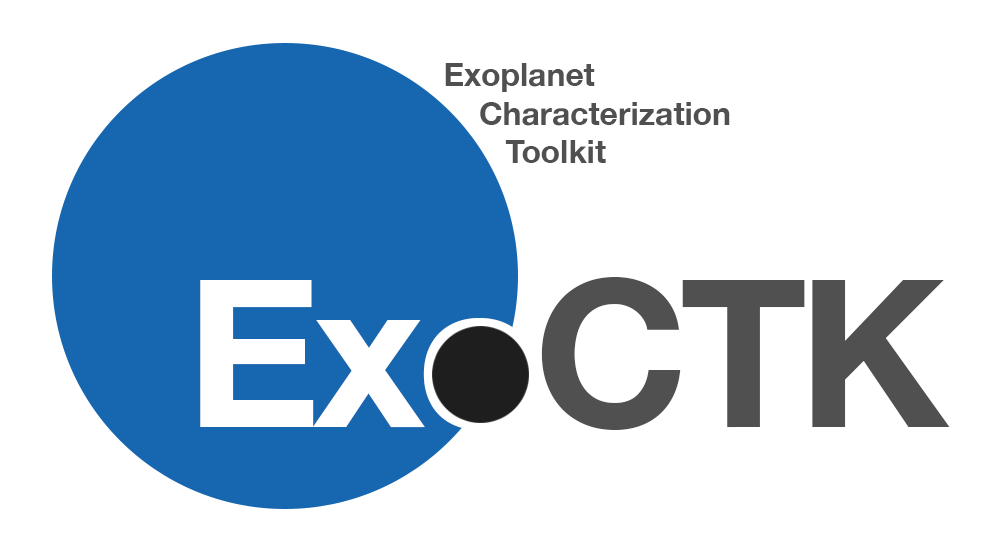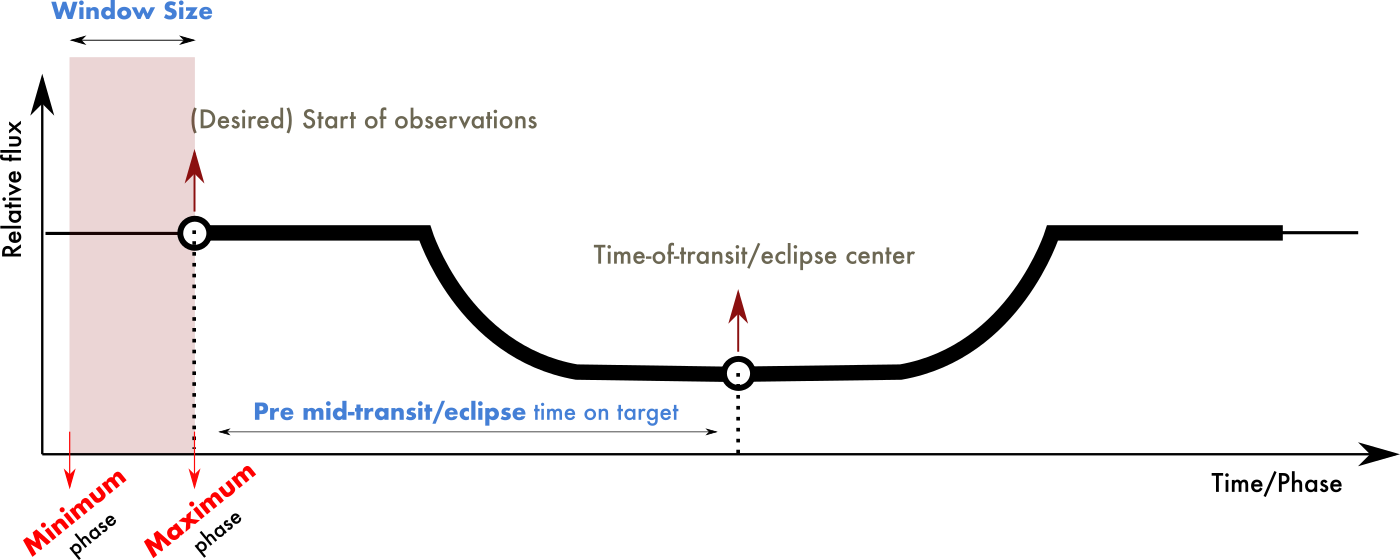# Phase Constraint Calculator

Due to uncertainties on the exact scheduling time of JWST observations, users are strongly encouraged to input minimum and maximum phases on the APT "Timing" requirements under the "Special Requirements" section when planning their observations. This gives the observatory a range of time (the "Window Size"; which should be of one hour or larger in order to not incur into direct scheduling overheads) on which observations might start. This is illustrated in the following diagram:The Phase Constraint Calculator provides a simple interface for calculating this JWST observation window for direct input on APT. This tool always assumes the zero-phase is at mid-transit, and thus that value is the one that should be input under "Zero phase (HJD)" in APT. Users can either resolve target properties from exo.MAST using the "Resolve Target" button below or input their own. If using the former, users are strongly encouraged to check that the retrieved properties are up-to-date.

The name of a target to resolve in ExoMAST

$$\small \text{Days}$$
Period of Target

(Only used to compute secondary eclipse time/phase constraints)

$$\small \text{Degrees}$$
Argument of periastron passage (only used to compute secondary eclipse time/phase constraints)

$$\small \text{Degrees}$$
Inclination of the orbit (only used to compute secondary eclipse time/phase constraints)

Calculate constraint for primary transits or secondary eclipses?

$$\small \text{Hours}$$
Time planned to be spent on target prior to mid-transit/eclipse (Default: 0.75 Hr + Max(1 Hr + T14/2, T14) when using Resolver)
$$\small \text{Hours}$$
Size of observation start window (Default 1 hour)

Calculate Phase Constraints for Target.

$$\small \text{BJD or HJD}$$
Time of transit-center of target (this goes under "Zero Phase" in APT). Automatically fills when hitting "Resolve Target".

Minimum Phase of Observation

Maximum Phase of Observation